Next: HEAT EQUATION EXAMPLE Up: What is Green's Function Previous: What is Green's Function

# FIN EQUATION EXAMPLE

Consider the steady temperature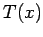in a fin of uniform cross section, which satisfies the following second order, linear, differential equation: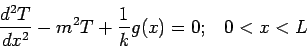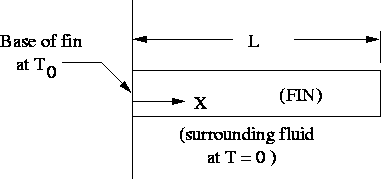Here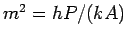where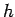is the heat transfer coefficient (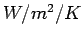),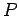is the fin perimeter (meters),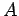is the cross section area of the fin (meters squared), and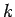is the thermal conductivity (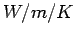). Quantity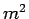describes heat loss by convection to a fluid at temperature zero from the sides of the fin (if the fluid temperature is at some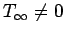you can always define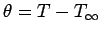to reset the fluid temperature to zero). Refer to the figure for the geometry. Term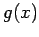represents volume energy generation within the fin (such as by electric heating or chemical reaction). The boundary conditions at the ends of the fin may be of several types, however consider the following specific boundary conditions: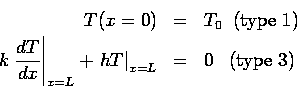The boundary at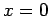is fixed at specified temperature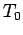and the boundary at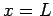sees heat loss by convection.

The temperature solution will be solved in terms of the Green's function, which is the response of the fin to a point source of heat. The GF for the above fin satisfies the following equations: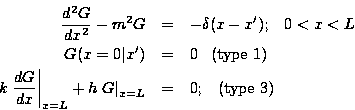Note that the above equations are similar to the temperature problem, except the energy generation term is replaced by a Dirac delta function and the boundary conditions are homogeneous. Most importantly, the boundary conditions must be of the same type as the specific temperature problem of interest. The Green's function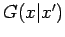represents the heat transfer response at observation point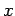to an infinitesimal heat source located at point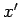.

The temperature solution is constructed from a suitable distribution of the GF within the body so as to reproduce the heating conditions. In this example, the temperature in the fin is given by: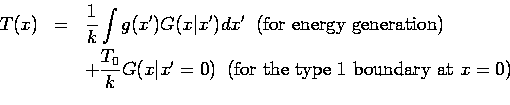The temperature solution contains an additive term for each non-homogeneous heating effect, in this case internal generation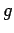and boundary temperature. There is no additive term needed for cooling effect atbecause this condition is homogeneous (temperature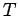appears in every term of the boundary condition). For a further discussion of steady heat conduction, see Green's function solution equation''.Next: HEAT EQUATION EXAMPLE Up: What is Green's Function Previous: What is Green's Function
2004-01-21